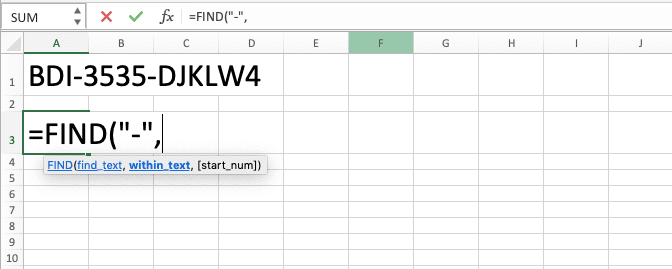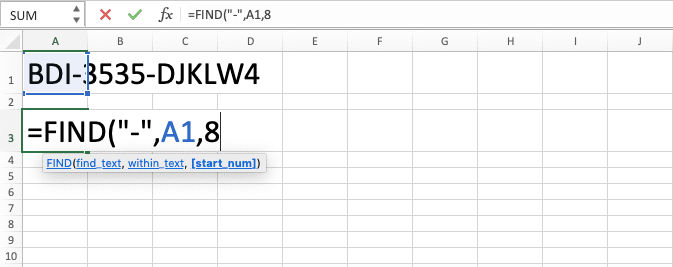How to Use the FIND Function in Excel: Usabilities, Examples, and Writing Steps - Compute Expert

# How to Use the FIND Function in Excel: Usabilities, Examples, and Writing Steps

Home >> Excel Tutorials from Compute Expert >> Excel Formulas List >> How to Use the FIND Function in Excel: Usabilities, Examples, and Writing Steps

In this tutorial, you will learn how to use the FIND function in excel completely.

When working in excel, we sometimes need to know the position of a data part in our data. We may need it for our further data processing or to get some results we need from our excel work. If we have already mastered how to use FIND function in excel, however, then we can get the position information easily.

Want to explore more about FIND and understand the way to use it properly in excel? Read this tutorial until its last part!

Disclaimer: This post may contain affiliate links from which we earn commission from qualifying purchases/actions at no additional cost for you. Learn more

## What is the FIND Function in Excel?

FIND function in excel is a function that helps you to find a data part position in your data. FIND is case-sensitive and doesn’t support wildcard characters in its input.

## FIND Usabilities

You can use FIND to get the position of a data part in data.

## FIND Result

FIND result is a number that represents the position of a data part in our data.

## Excel Version from Which We Can Start Using FIND

We can start using FIND since excel 2003.

## The Way to Write It and Its Inputs

Here is the general writing form of a FIND function in excel.

= FIND ( data_part_to_find , data , [ starting_position_to_find_the_data_part ] )

And here is a bit explanation of the inputs we need to give when we write FIND.
• data_part_to_find = the data part we want to find the position of in our data
• data = the data where we want to find the position of a data part
• [starting_position_to_find_the_data_part] = optional. The starting position in the data where we want to start looking for the data part position that we want. The default input is 1 or from the most left position of the data

## Example of Its Usage and Result

You can see the usage and result example of FIND below.In the example, you can see how we use FIND to find the data part position we want in our data. Just input the data part you want to find and the data you want to find the data part from. Input the starting position to find the data too if you need it (in most cases, you input it when the data part you want to find has multiple occurrences in your data).

FIND is case-sensitive as it will find the position of the data part we want in its exact letter case. You can see this FIND nature in action clearly in the second row of the example.

## Writing Steps

Need guidance to write your FIND formula in excel? Take a look at its writing steps in detail below!

1. Type an equal sign ( = ) in the cell where you want to put the FIND result2. Type FIND (can be with large and small letters) and an open bracket sign after =3. Input the data part that you want to find the position of in your data. Then, type a comma sign ( , )4. Input the data from where you want to find the data part position5. Optional: Type a comma sign. Then, input a number that represents the starting position from where you want to find the data part in your data. If you don’t input anything here, then FIND will try to find the data part from position 1 (the most left position).6. Type a close bracket sign7. Press Enter
8. Done!## FIND vs SEARCH

You may have already known about the existence of the SEARCH formula before in excel. It is a formula you can also use to find the position of a data part in your data in excel.

Then, what is the difference between if we use FIND and if we use SEARCH? Well, there are two crucial differences we should be aware of.

The first one is their case-sensitivity. While FIND is case-sensitive, SEARCH isn’t.

The second one is their treatment of wildcard characters. While FIND doesn’t support them, you can use wildcard characters in your SEARCH input.

Here is an implementation example of both formulas in excel which shows their differences clearly.You can see in the example how FIND and SEARCH can be similar or different in their results. When there is no difference whether we are case-sensitive or not when finding our data part, their results are similar.

However, as you can see in the second implementation there, FIND and SEARCH give different results when case-sensitivity matters. FIND pays attention to the letter cases while SEARCH doesn’t. That is why FIND produces 14 there (the first “E” instance position from the fifth position in the data) while SEARCH produces 5 (the first “e” or “E” instance position from the fifth position in the data).

We can also see their differences in the third implementation example. If we use wildcard characters in our data part input (* or ?), then SEARCH can process it while FIND can’t. FIND will produce an error if it doesn’t find a literal * or ? in the data.

## Exercise

After you have understood FIND and how to use it properly in excel, now let’s do an exercise. This is so you can master the practice of using FIND in excel better!

### Questions

1. What is the position of the fourth “_” sign in the index code?
2. What is the position of 4562 in the index code?
3. Try to take the 11021990 number from the index code by using MID combined with FIND! (This question might be a bit harder to answer. Learn MID first here if you haven’t mastered the basics of the formula yet!)

You cannot use FIND to get the position of date or time data part in excel. That is because date and time data real form in excel is a number. When you use FIND on a date or time data, excel will take the position of that number part instead.

If you really need to use FIND on your date or time data, you should convert the data into text first. By doing that, FIND can get the position of the date or time data part you need!

Related tutorials you should learn too:

Get updated excel info from Compute Expert by registering your email. It's free!